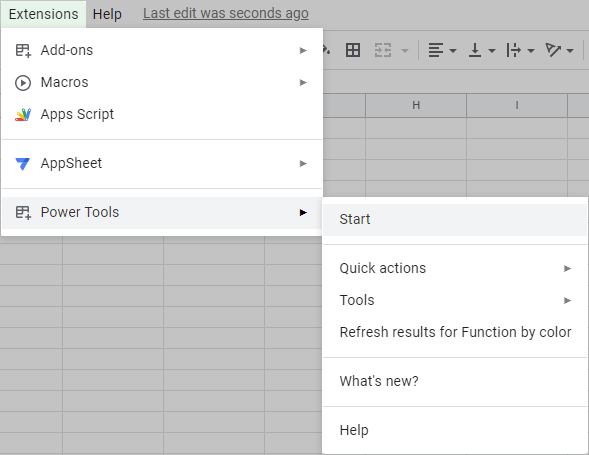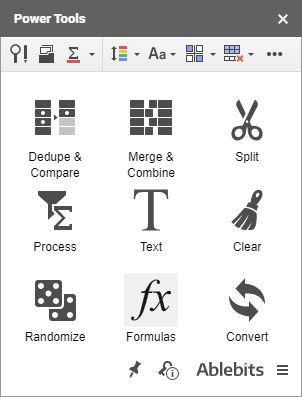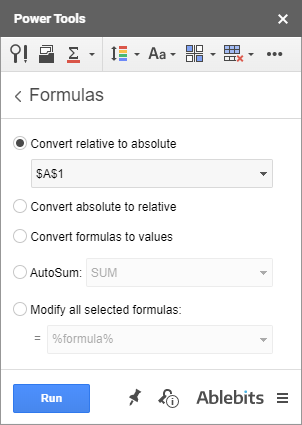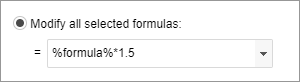# Change multiple formulas in Google Sheets

Power Tools provides you with various utilities to speed up most of your daily tasks. The Formulas tool will help you change the formula type, get values instead of the formulas in the range, or perform quick calculations for all columns or rows.

## How to convert formulas and auto apply functions to all selected columns2. Click on the Formulas icon on the add-on sidebar:3. Select the range of cells you want to work within and pick one of the following options:• Convert relative to absolute
Select this radio button to change the type of formulas in the selected range. The drop-down list lets you choose between three kinds of absolute references to convert formulas to:

• Absolute cell reference — \$A\$1
• Absolute column reference — \$A1
• Absolute row reference — A\$1
• Convert absolute to relative
Choose this option to convert absolute references in the formulas to relative.
• Convert formulas to values
Get the calculation results or values instead of the formulas used in the selected range.
• AutoSum
This one will automatically add formulas under each selected column with numbers.

Choose cells under all columns where you want to insert totaling formulas and pick the necessary function from the list.

Tip. If you select a blank column to the right of your table with numbers, the tool will calculate all values in each row.
Tip. The add-on offers the most common functions to choose from: Sum, Count, Counta, Average, Averagea, Min, Max, Product, Mode, Stdev, Var, Median.
• Modify all selected formulas
Pick this option to transform your calculations but keep all formulas where they are.

Simply enter additional calculations before or after the formula in the edit field, where %formula% stands for your current formulas in the selected range:Click Run to change all formulas in the selected range at once according to the option you select.

### ResponsesHi, so I am trying to convert *part* of the formula to absolute, but keep the rest relative.

The Equation i am working with it =IMSUM(IMPRODUCT(B2,B2),B2), and I want to convert the last B2 to absolute, without converting the other B2s. but the convert tool converts the whole formula. is there a way around this?Katerina Bespalaya says:
November 13, 2019 at 3:23 pm

Hi Calder,

Unfortunately, our tool can't convert only one of the cell references in your formula to absolute.Any help would be GREATLY appreciated.

I need to have a certain cells in a row autosum in the column named "Total Estimated Expenses" and have new rows do the same thing automatically as they are added.Hello Mark,Andrew Serafim says:
January 28, 2020 at 5:55 pm

So I have a large range of cell reference formulas and I am trying to convert them all at the same time from relative to absolute.

When I select them all and then use the relative to absolute function none of them actually are being converted to absolute references.

Any help or explanation would be appreciated, otherwise I have to go in manually and adjust them all, which will take forever.